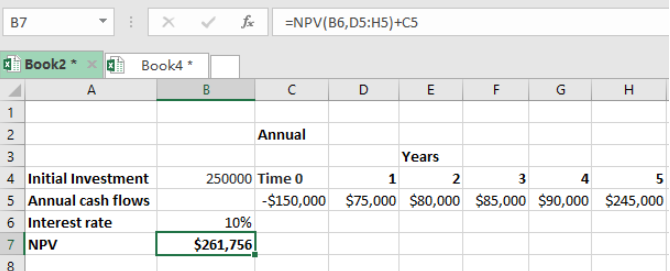Get instant live expert help with Excel or Google Sheets“My Excelchat expert helped me in less than 20 minutes, saving me what would have been 5 hours of work!”

#### Post your problem and you’ll get expert help in seconds.

Your message must be at least 40 characters
Our professional experts are available now. Your privacy is guaranteed.

# An Overview of the NPV Function in Excel

• The net present value, or simply NPV is the present value or actual value of a future cash flow. In other words, the NPV refers to the value of money that we are anticipating in the future. In many financial institutions, it is important to understand the value of future cash flows. To understand the value of future cash flows, we need to know the discount rate.
• Also, we need to know that when calculating net present value, we consider both the inflows as well as out flows. In flows in this case refers to the profits that we will be getting from a given investment. Out flows refers to any expenditures, including the amount of money used as the initial investment.
• It is important to know that we can get either a positive or negative NPV.
• A positive NPV means that total incomes from the investment is higher than total expenditures. This implies that the investment is actually a good one.
• When we get a negative NPV, it implies that the investment is not worth it, as the total expenditures are more than total income from the investment.
• Total discounts are discounted using a given discount rate which correspond to the best alternative investment.Figure 1. Final result

## General syntax of the formula

`=NPV(rate, cash flows) + Initial investment`

Note that the initial investment is a negative, as it is a cash out flow.

Excel function NPV anticipates the discount rate to be annual. So if you have the period in other measurements such as monthly, you need to adjust it to fit.

## Instant Connection to an Expert through our Excelchat Service

Most of the time, the problem you will need to solve will be more complex than a simple application of a formula or function. If you want to save hours of research and frustration, try our live Excelchat service! Our Excel Experts are available 24/7 to answer any Excel question you may have. We guarantee a connection within 30 seconds and a customized solution within 20 minutes.

Solution examplesI have a figure which is the sum of 9 months of payments. Each payment decreases by the same amount (X) so the first payment is Y, second payment is Y-X, third is Y-(Xx2), forth is Y-(Xx3) and so on. I am trying to calculate what each of the 9 payments should be.
Solved by A. W. in 60 mins